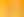Hunting for Patterns and Rules

# Stages1. Functions and Relations

EXPLORE

Suggested Learning Intentions

• To understand that functions describe the relationships between numbers
• To understand that a relation is a set of inputs and outputs
• To understand that a function is a relation with one output for each input

Sample Success Criteria

• I can explain that the relationship between one number and another number is described by the functions performed on them
• I can articulate that a relation is a set of inputs and outputs
• I can determine the output of a number given a known input
• I can demonstrate the effect of a function being performed using a variety of manipulatives
EXPLORE

2. Exploring Patterns

EXPLORE

Suggested Learning Intentions

• To understand that a pattern is a predictable arrangement of elements
• To understand that patterns are determined by the application of different functions
• To understand the difference between repeated and growing patterns

Sample Success Criteria

• I can identify and describe a pattern
• I can model a pattern using a range of different manipulatives
• I can continue a pattern using appropriate functions (operations)
• I can create a pattern using a variety of functions (operations)
• I can articulate what a repeated pattern is and what a growing pattern is
EXPLORE

3. Equivalence

EXPLORE

Suggested Learning Intentions

• To understand that expressions on either side of an equal sign must be balanced
• To explain that when a function on one side of an equation is performed an equal function must be performed on the other side to maintain balance

Sample Success Criteria

• I can articulate that an equals sign represents a balance of the expressions on either side of it
• I can create equivalent number sentences/equations
• I can manipulate equations or number sentences to maintain the balance on both sides
• I can model my problem solutions using a variety of manipulatives
EXPLORE

4. Using Symbols to Recognise Unknowns

EXPLORE

Suggested Learning Intentions

• To understand that symbols (pronumerals) can be used to represent a variable or an unknown quantity in an equation
• To understand that symbols can be used to represent an unknown in a pattern or a generalisation
• To understand that these equations can be manipulated to determine the unknown quantity

Sample Success Criteria

• I can use a symbol (pronumeral) to represent an unknown in an equation
• I can use a symbol (pronumeral) to represent an unknown in a pattern
• I can manipulate equations to determine the unknown (symbol/pronumeral)
• I can justify my thinking using other representations of the mathematics
EXPLORE